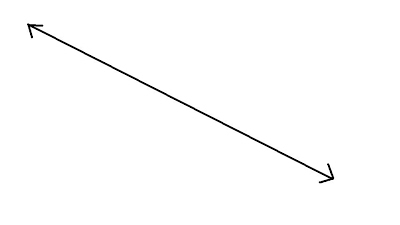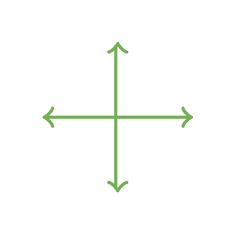Multiplication
Geometry
Fractions
Mental Math
Math Mystery
100

10 x 58=

580

100What is this called?

A line

100

1/4 + 2/4=

3/4

100

10 x 11=

110

100

What is the answer to a multiplication problem called?

Product

200

900 x 9=

8100

200

A right angle is formed at ___ degrees?

90 degrees

200

Change to an improper fraction.

2 1/8

17/8

200

200 x 2 =

400

200

What is the answer to a division problem called?

Quotient

300

110 x 100

11,000

300
What is the angle called that is less than 90 degree?

Acute

300

Change this improper fraction into a mixed number.

6/3

2

300

120 + 120 + 20 + 20 + 1=

281

300

Joshua arrived to work at the dog toy factory at 5:50 a.m. He stayed at the factory for 5 hours and 10 minutes.  What time did Josh leave the factory?

11:00 am

400

Name 4 factors of 32

1, 2, 4, 8

400What do you call this image?

perpendicular lines

400

Timmy ate 1 1/2 onions before he read his book, and ate another 2 1/2 onions afterward. How many onions did he eat?

4 onions

400

4 times 15

60

400

To find the area of a rectangle you have to multiply _____ x ______

Length x Width

500

Name 2 multiples of 15

30, 45, 60, 75, 90, 105....
500

What do you call an angle that is greater than 90 degrees?

Obtuse

500

5 1/8 + 4 2/8 =

9  3/8

500

(4 x 6) + (5 x 4 )

44

500

To find perimeter of a shape you have to.....

Add up the length of all the sides

Click to zoom